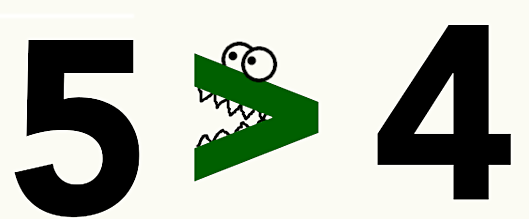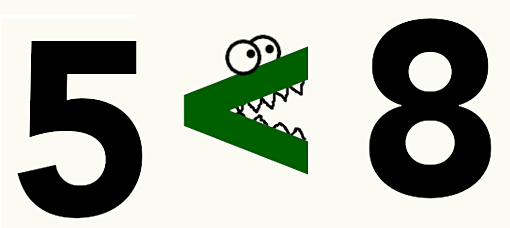In mathematics, economics, physics, or other subjects that involve calculations, you must have encountered the sideways carat symbols. What exactly do they signify? These little signs are inequalities symbols. But the most notable thing about them is that students find them very hard to remember because they appear similar. Notably, equality symbols are some of the most important signs because they help demonstrate the relationship between equations and numbers.

In this post, we are going to dig deeper into the greater than less than signs to demystify how they work and assist you in remembering them.## What Does Greater and Less than Symbol Mean?

The two signs are used for comparing two things. In math problems, the inequality signs do not help solve them (you do not get a distinct answer). Rather, they show how a number is related to another by indicating that it is greater or less. So, you can tell one thing when the < greater than or less than signs are used: the numbers under consideration are not equal. To show you the inequality symbols, we will present them in a chart. Have a look:

 Symbol Symbol meaning < This symbol means less than. It means that the number provided on the left is less compared to the one on the right. For example, 4<5. > This symbol means greater than. It means that the number on the left side is bigger compared to the one on the right. Here is an example; 10>9. ≤ This symbol means that the number provided is less than or equal to the one on the left. Here is an example; 4 or 5 ≤ 4. ≥ This sign indicates that the number on the right side is greater or equal to the one on the left. Example, 5 or 6 ≥ 5. ≠ This symbol simply means does not equal. The number on the left side does not equal the one on the right. Example, 4 ≠ 5.

### The Secret to Remembering < Greater Than And Less than Symbol

Now that we have demonstrated what less than greater than or equal to signs mean, we will now turn to the methods that you can use to remember them.

• Using alligator method

This is one of the recommended options to help you easily remember less than and greater than symbols. Well, you simply take the symbols as alligators, while numbers on either side are fish counts. Because the alligator always tries to eat fish, the number the mouth is open towards is always greater than.In the example above, the alligator’s mouth is open towards the five. Therefore, five is greater than four. The method can also help to tell you about the other number. If five is greater than four, it means that four is less than five.In this case, consider five to be a small alligator’s mouth that is about to catch and eat some fish. Because the mouth is pointed towards eight, it implies that eight is greater than five. The sign will always tell you how the two numbers are related. So, if eight is bigger than five, it means that five is also less than eight.

To make remembering more than less than signs, be creative. Go ahead and customize the alligator. For example, add some eyes to indicate where the alligator is facing.

• L- Sign method

If you take the less sign and rotate a little, what do you get? An L sign. This trick is rather simple; simply tell your mind that less than symbol commences with letter L. Therefore, the sign that looks like an L sign should mean less than. For example, since > does not resemble an L, it cannot be less than. However, < will make an L with simple tilting. Therefore, it is the less than sign.

• Remembering the equal sign

Once you have grasped the concepts of the L method and the Alligator method, things will start becoming easy. The equal to or less than and equal to signs simply feature a half equal sign symbol under them. Take the example, 5 or 6 ≥ 2. This equation shows a greater sign, but it also has a half equal sign. Therefore, it implies that either five or six are greater or equal to two.

If you put it the other way, it will also be true. However, the situation will be less than or equal to a type of relationship.

When you use the “does not equal sign,” remembering it is even simpler. You simply check the equal sign that has been crossed. Take the example 2 ≠ 4. In this case, it simply means that two is not equal to four. The number on the left is not equal to the one on the right.

### Tricks to Use When Working with Less than and Greater than signs

Notably, many inequalities can be tricky because you do not solve for a straightforward answer. Therefore, you can find them easier to work with by keeping the following in mind:

• Inequalities are used to demonstrate relationships.
• Always isolate the variables you are working with to know which one is greater than, less than, equal to or not equal.
• When you use the negative numbers, the effect is changing the greater or less signs.
• In most cases, avoid multiplying or dividing by a variable.

### Now You Know Less and Greater than Sign … What Next?

In this post, we have demonstrated less than and greater than signs. So, the next thing is to apply them in your studies. Try to identify the signs and solve related problems. It should be easy, right? But if you find it still complex, don’t waste your time thinking that homework is too hard for you and should be banned – it is time to seek professional writing help with it.

Consider working with professional writers because they understand every concept in mathematics and are willing to help. Indeed, they can help you do all types of papers, including maths papers, geography term papers, law essays, coursework, and any other type of assignment.